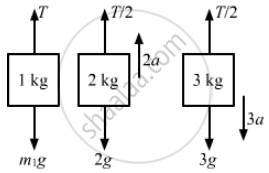Advertisement Remove all ads

# In the Previous Problem, Suppose M2 = 2.0 Kg and M3 = 3.0 Kg. What Should Be the Mass M, So that It Remains at Rest? - Physics

Sum

In the previous problem, suppose m2 = 2.0 kg and m3 = 3.0 kg. What should be the mass m, so that it remains at rest?

Advertisement Remove all ads

#### SolutionFor m1 to be at rest, a1 = 0.
m1g = 0
T = m1g    ...(i)
For mass m2,
T/2 − 2g = 2a
T = 4a + 4g    ...(ii)
For mass m3,
3g – T/2= 2a
T = 6g − 6a    ...(ii)

From equations (ii) and (iii), we get:
3T – 12g = 12g – 2T
T = 24g/5= 4.08g
Putting the value of T in equation (i), we get:
m1 = 4.8kg

Is there an error in this question or solution?
Advertisement Remove all ads

#### APPEARS IN

HC Verma Class 11, 12 Concepts of Physics 1
Chapter 5 Newton's Laws of Motion
Q 29 | Page 81
Advertisement Remove all ads
Advertisement Remove all ads
Share
Notifications

View all notifications

Forgot password?